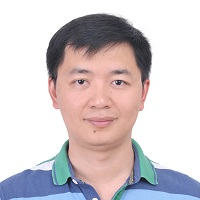A Novel Numerical Approach to Time-Fractional Parabolic Equations with Nonsmooth Solutions
Speaker
Prof. Dong-Fang Li
School of Mathematics and Statistics, Huazhong University of Science and Technology
Abstract

This talk is concerned with construction and analysis of some novel numerical methods for time-fractional parabolic equations. Due to the Caputo time derivative being involved, the solutions of equations are often singular near the initial time t = 0 even for a smooth setting. Based on a simple change of variable, an equivalent s-fractional differential equation is derived and a novel L1 finite difference method is proposed for solving the s-fractional differential equation. Under the proved regularity, we show that the proposed L1 method provides the optimal accuracy. Numerical examples for both linear and nonlinear fractional equations are presented in comparison with classical L1 methods on uniform meshes and graded meshes, respectively. Our numerical results clearly show the accuracy and efficiency of the proposed methods.